# RC circuit differential equations

• Engineering
evol_w10lv

## Homework Statement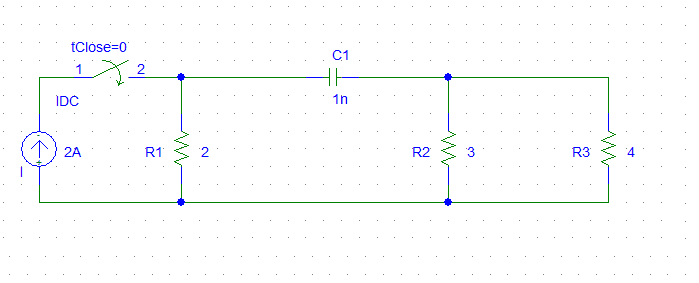Task is to write differential equation for this circuit.

## The Attempt at a Solution

I'll try to solve the task, but now I want to know, is it possible to use voltage source instead of current source. For example, I can calculate ekvivalent resistance R_1, R_2 an R_3 parallel. Then current source * ekvivalent (total) resistance = voltage source?
Like this: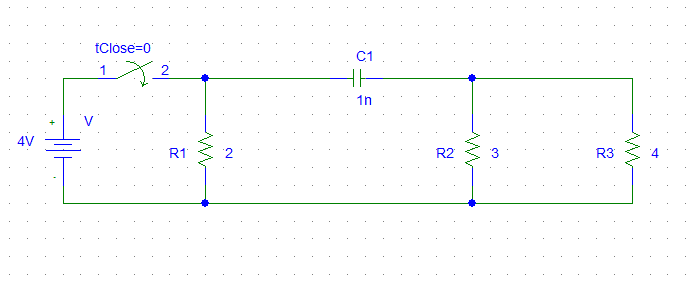Don't look at numbers, they are random.. I just want to know about idea.
If it's possible, then I can use V(Thevenin) and R(Thevenin).

OR:
I can calculate R(Thevenin) = (R2*R3)/(R2+R3), I guess.
But I don't know, how to calculate I(Thevenin). Or I have to use Northon method, when we've got capacitor?

Can you suggest something?

Mentor
In your original circuit the current source and first resistor form a Norton equivalent when the switch is closed. No reason why you couldn't turn that into a Thevenin equivalent.

Be sure to take into account the circuit properties affected by the switch if you need to determine the circuit operation if the switch is re-opened at some future time.

evol_w10lv
Do you mean like this: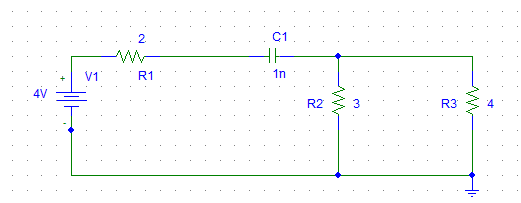?

And then again I need to change circuit to Thevenin equivalent as I did before with RL circuits?

Last edited:
Mentor
Yes and yes, in your figure you can absorb R2 and R3 into the Thevenin model.

evol_w10lv
Then:
R(Thevenin) = ((R2*R3)/(R2+R3)) + R1
E(Thevenin) = E = I*R1

(((R2*R3)/(R2+R3)) + R1)*C*dUc/dt + Uc = E

Is it correct?

Mentor
Yup. Looks good.

evol_w10lv
Easier than I thought. But which initial condition I have to use? From picture in my first post?
But there is Ic(0), but here I need Uc(0). Seems to me, that I don't understand, how to find intial condition.

Mentor
You can use the initial conditions for your "new" circuit. If the capacitor begins with no charge then its initial potential is zero. It will "look like" a short at time t = 0, so you can find the initial current, too.

evol_w10lv
Ic(0) = 0 and Uc(0)= E ?
Anyway, I'll try to finish all calculations tomorrow. It's to late today in my country.

Mentor
Ic(0) = 0 and Uc(0)= E ?
Anyway, I'll try to finish all calculations tomorrow. It's to late today in my country.

With the Thevenin circuit, when the switch closes there will be a closed loop. The capacitor will look like a short, so just the Thevenin resistance is seen as the load at time t = 0.

evol_w10lv
It means that there is no voltage through the capacitor, so Uc(0)=0.

Mentor
It means that there is no voltage through the capacitor, so Uc(0)=0.

"Across". Voltage is "across" a component, current goes "through". But yes, that's the right idea.

evol_w10lv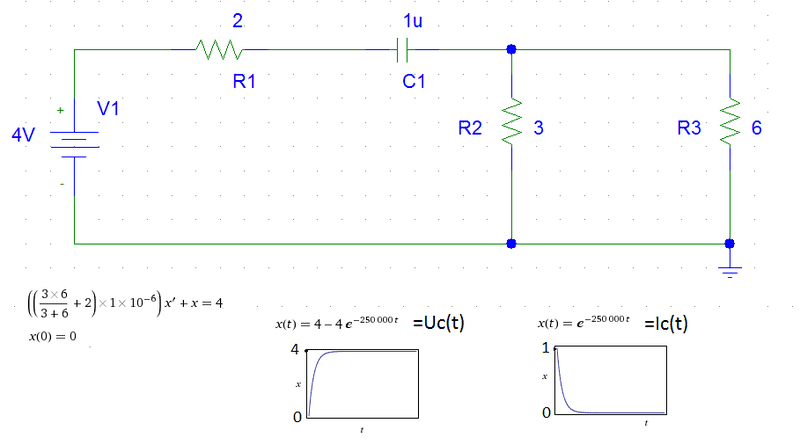And it is same as solution for this: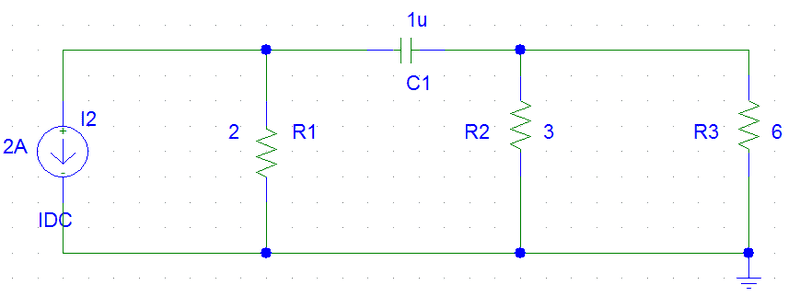Mentor
One difference between the two is that the current will be flowing in opposite directions and the potential across the capacitor will have the opposite polarity. To make them identical, change the polarity of either the current or voltage source.

evol_w10lv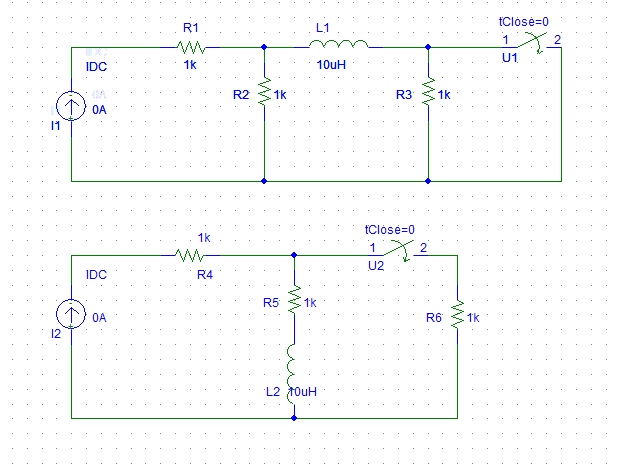How to redraw circuit here, when current source and resistor are in series? I guess, here we can't use Norton because resistor and current source not parallel.

Mentor
How to redraw circuit here, when current source and resistor are in series? I guess, here we can't use Norton because resistor and current source not parallel.

Big tip: A resistor in series with an ideal current source has no effect on the circuit! The current source will produce its specified current no matter what is in its path. It won't even contribute to Norton/Thevenin resistances for the circuit.

•1 person
evol_w10lv
Tried to solve second one (from post #15) with two methods: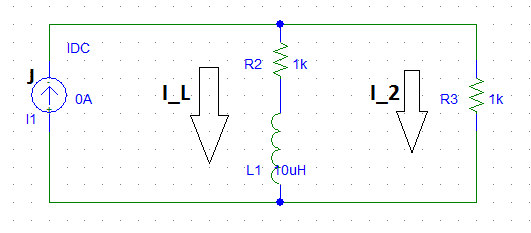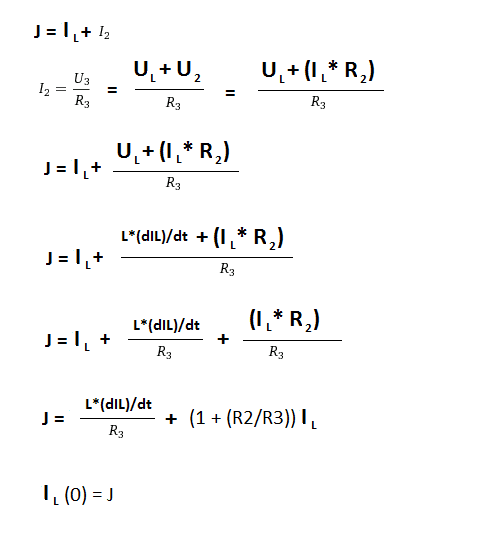And method 2: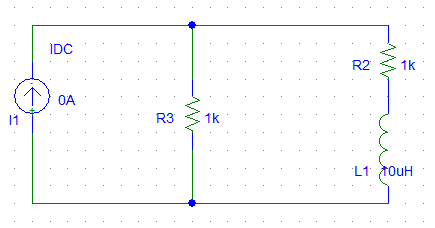Then like before I used Norton/Thevenin equivalent: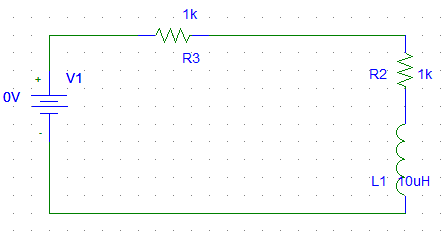E = R3 * J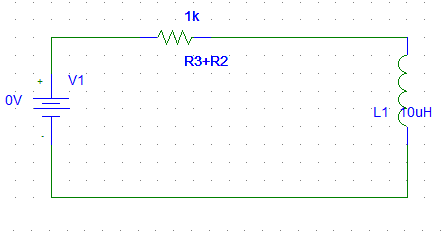E(Thevenin) = E = R3*J
R(Thevenin) = R2 + R3

E = L*dIL + (R2+R3)*IL

Actually the first circuit (from post #15) is simular, but why there are switch without anything? Is there something tricky in the solution?

Last edited:
Mentor
Actually the first circuit (from post #15) is simular, but why there are switch without anything? Is there something tricky in the solution?
The switch changes the circuit by eliminating R3 when it closes (it shorts it out). So there will be a different steady-state situation for each position of the switch.